The method given in Section 2.2.1 specifies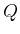as a First-In First-Out (FIFO) queue, which selects states using the first-come, first-serve principle. This causes the search frontier to grow uniformly and is therefore referred to as breadth-first search. All plans that havesteps are exhausted before plans with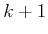steps are investigated. Therefore, breadth first guarantees that the first solution found will use the smallest number of steps. On detection that a state has been revisited, there is no work to do in line 12. Since the search progresses in a series of wavefronts, breadth-first search is systematic. In fact, it even remains systematic if it does not keep track of repeated states (however, it will waste time considering irrelevant cycles).
The asymptotic running time of breadth-first search is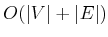, in which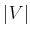and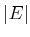are the numbers of vertices and edges, respectively, in the state transition graph (recall, however, that the graph is usually not the input; for example, the input may be the rules of the Rubik's cube). This assumes that all basic operations, such as determining whether a state has been visited, are performed in constant time. In practice, these operations will typically require more time and must be counted as part of the algorithm's complexity. The running time can be expressed in terms of the other representations. Recall that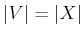is the number of states. If the same actions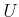are available from every state, then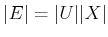. If the action sets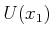and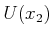are pairwise disjoint for any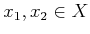, then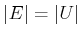.